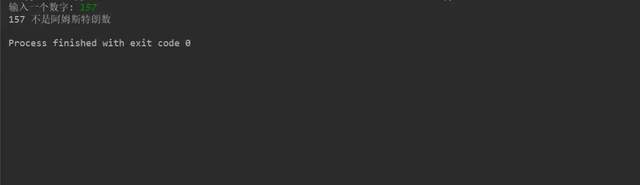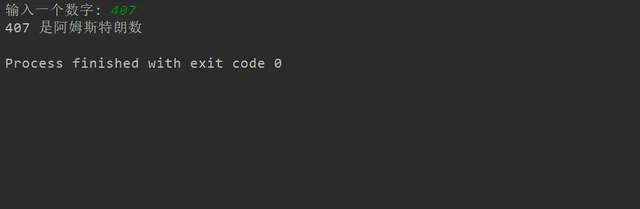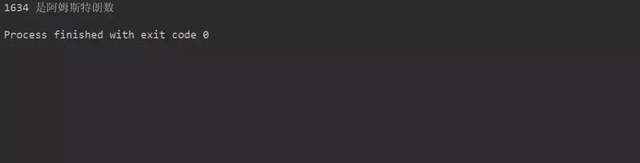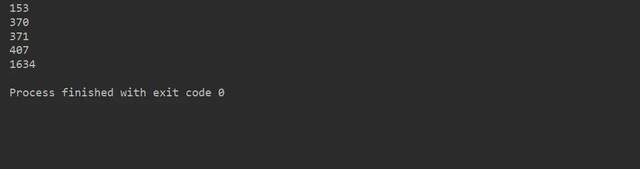一、什么是阿姆斯特朗数？

abcd... = an + bn + cn + dn + ...

153 = 1*1*1 + 5*5*5 + 3*3*3  // 153是一个阿姆斯特朗数。

1. 检查阿姆斯特朗数（3位数字）

# 检查该数字是否为阿姆斯壮数字的Python程序

# 接受用户的输入
num = int(input("输入一个数字: "))

# 初始化sum
sum = 0

# 求出每个数字的立方和
temp = num
while temp > 0:
digit = temp % 10
sum += digit ** 3
temp //= 10

# 显示结果
if num == sum:
print(num,"是阿姆斯特朗数")
else:
print(num,"不是阿姆斯特朗数")2. 检查是阿姆斯特朗的n位数字

num = 1634

# 将num变量更改为string
# 并计算出长度（位数）
order = len(str(num))

# 初始化 sum
sum = 0

# 求出每个数字的立方和
temp = num
while temp > 0:
digit = temp % 10
sum += digit ** order
temp //= 10

# 显示结果
if num == sum:
print(num, "是阿姆斯特朗数")
else:
print(num, "不是阿姆斯特朗数")3. 在整数中查找阿姆斯特朗数

# Python程序在整数中查找阿姆斯特朗数

lower = 100
upper = 2000

for num in range(lower, upper + 1):

# order 个数
order = len(str(num))

# 初始化 sum
sum = 0

temp = num
while temp > 0:
digit = temp % 10
sum += digit ** order
temp //= 10

if num == sum:
print(num)三、总结

IT共享之家——————- End ——————-